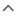# Fuel Money Calculator In Malaysia

Car Loan Calculator
Insurance Calculator
Fuel Cost Calculator
Select Cars
Fuel Type
Fuel Consumption (L/100km)
Fuel Price (RM)
RM
Year Distance (km)
• ### What is the fuel price in Malaysia today?The latest fuel price in Malaysia today, you can click here to view: petrol price Malaysia

• ### How do you calculate fuel cost?First, divide the distance of the route by your miles per litre figure to discover how many litre of petrol you will need, then multiply the number of litre by the price of fuel. For example 60L/695KM*100=8.63L/100KM. If you know the price of fuel, then you can simply multiply the price per litre by the result and that gives you your cost per 100km.

• ### How do I calculate fuel consumption per 100km?To calculate fuel consumption per 100 km, divide the amount of fuel used in liters by the distance in kilometers and multiply the product by 100.

Yearly Fuel Payment
TBC